# Community

Blog Events Webinars Tutorials Forum
×
Community Blog GCC: Graph Contrastive Coding for Graph Neural Network Pre-training

# GCC: Graph Contrastive Coding for Graph Neural Network Pre-training

This article introduces Graph Contrastive Coding (GCC), pre-training framework that uses the contrastive learning method to pre-train graph neural networks.

Download the "Cloud Knowledge Discovery on KDD Papers" whitepaper to explore 12 KDD papers and knowledge discovery from 12 Alibaba experts.

By Jiezhong Qiu, Qibin Chen, Yuxiao Dong, Jing Zhang, Hongxia Yang, Ming Ding, Kuansan Wang, Jie Tang

Graph representation learning has received extensive attention. However, most graph representation learning methods are used to learn and model graphs for domain specific problems. The generated graph neural networks are difficult to transfer out of the domain. Recently, pre-training has been a great success in many domains, which significantly improves the model performance in downstream tasks. Inspired by Bidirectional Encoder Representations from Transformers (BERT, Devlin et al., 2018), we began to study the pre-training of graph neural networks, hoping to learn the general graph topology features. We design Graph Contrastive Coding (GCC), a graph neural network pre-training framework that uses the contrastive learning method to learn the intrinsic and transferable structure representations. This paper "GCC: Graph Contrastive Coding for Graph Neural Network Pre-training" has been accepted by KDD 2020 Research Track.

Traditional graph representation learning primarily uses skip-gram based word representation learning models such as DeepWalk and Node2vec in natural language processing (NLP). These methods model the similarities between neighboring nodes, and the created models and trained representations are not generic but limited to specific networks. Different from these traditional attempts, GCC focuses on structural similarities. The representations learned by GCC are generic and can be transferred to all types of networks. The following figure shows the basic framework of GCC.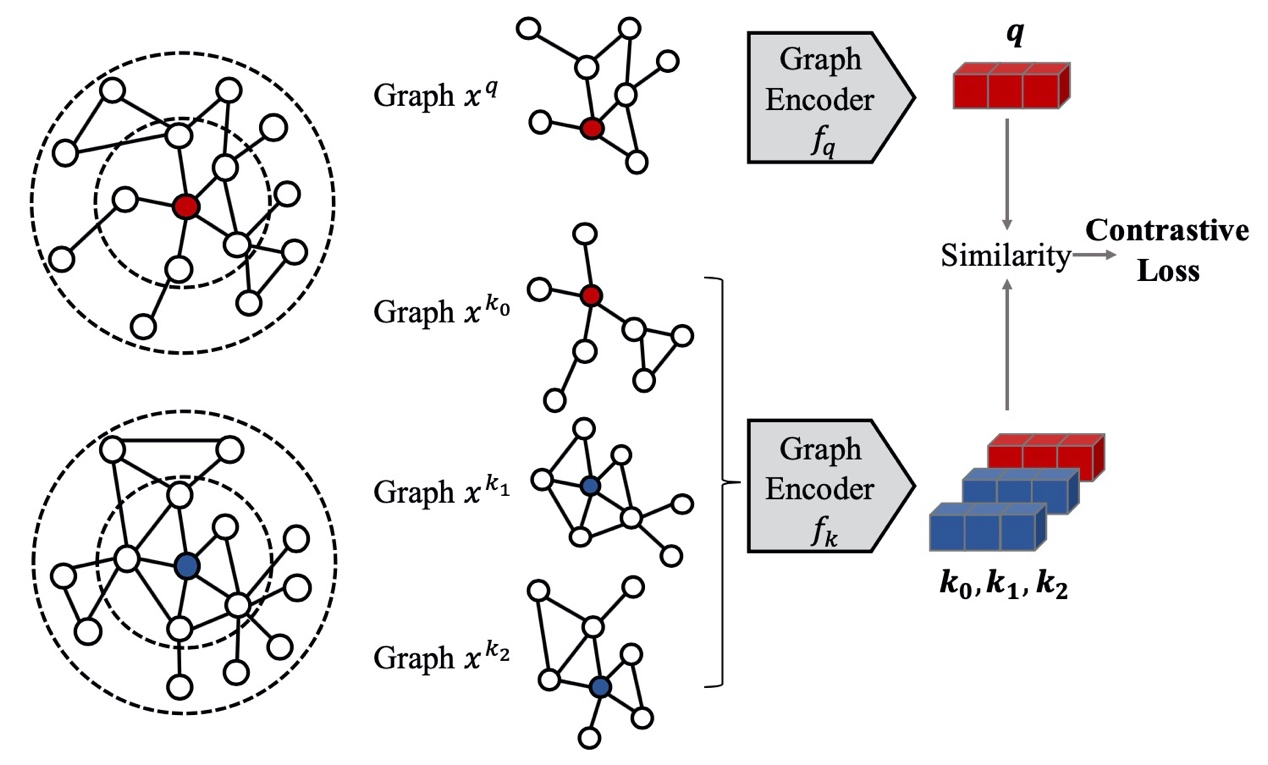GCC leverages the contrastive learning framework to reduce the spatial distance between positive sample representations and sample representations while increasing the spatial distance between negative sample representations. In contrastive learning, for a given query representation q, a candidate set contains K+1 representations {k_0,k_1,⋯,k_K}, of which k_+ are positive samples. The loss function is optimized as follows: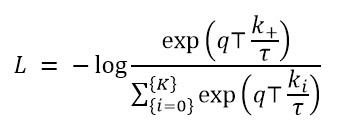q and k are low-dimensional representations of the samples `x^q` and `x^k`, respectively. GCC is mainly designed to sample the subgraph of the network (or r-ego network) that is formed by the r-order neighbors of a specific node. Positive samples are the networks sampled from the same r-ego network, whereas a large number of negative samples are the subgraphs sampled from other r-ego networks. After acquiring both positive and negative samples, we started to build a graph encoder for graph representation learning. Any graph neural network can be used as a GCC encoder. In practice, we used a graph isomorphism network (GIN) as the encoder.

In contrastive learning, it is required to maintain the K-size dictionary and encoders. Ideally, to calculate the preceding loss function, the dictionary should cover all negative samples. This makes K extremely large and the dictionary difficult to maintain. To ensure model efficiencies, we added Momentum Contrast (MoCo, He et al., 2020). In the MoCo framework, we need to maintain a queue of negative samples in order to increase the dictionary size K. These negative samples are derived from the samples of previously trained batches. For the θ_q parameter of the f_q encoder of q, updates are propagated in reverse order. For the θ_k parameter of the f_k encoder of k, updates are propagated as follows: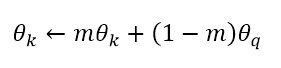m denotes the momentum, which is specified by a hyperparameter. For GCC, MoCo is more efficient than other methods.

GCC is applicable to downstream tasks at the graph level and node level. Downstream tasks at the graph level can be input as subgraphs. This situation is the same as that in pre-training. For downstream tasks at the node level, we need to acquire their r-ego networks or subgraphs sampled from their r-ego networks.

To verify the effect of GCC, we conducted a series of experiments, including node classification, graph classification, and similarity search. The results are as follows: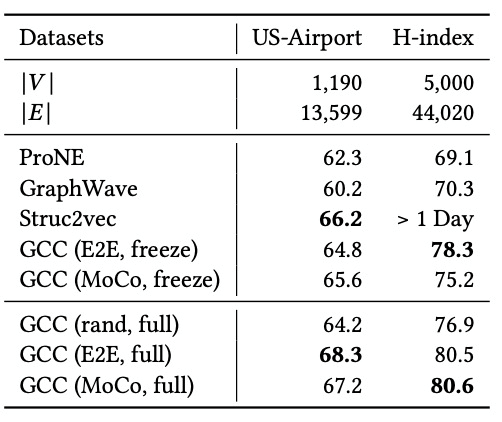Node classification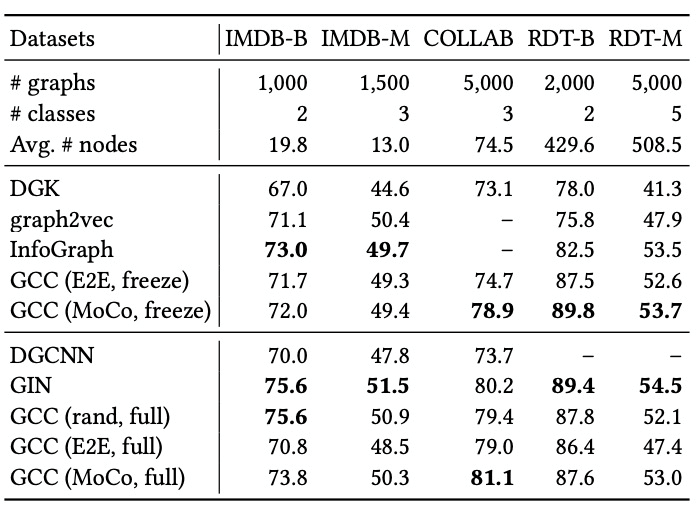Graph classification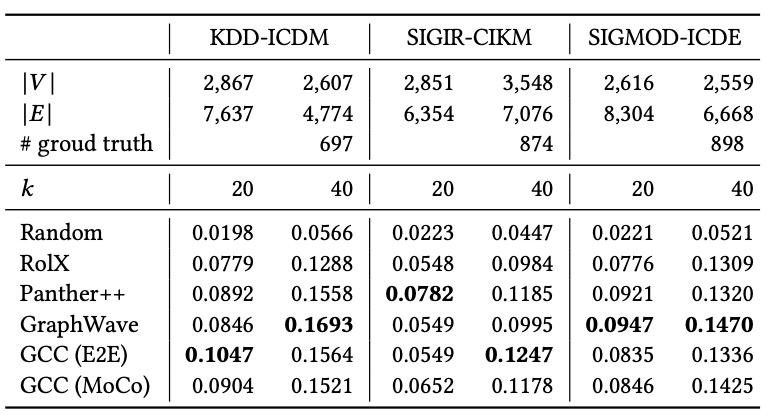Similarity search

According to the experiment results, GCC excels at processing multiple tasks and datasets. Its performance is close to or better than the existing optimal model. This shows the effectiveness of GCC.

To sum up, in this work, we propose GCC, which is a graph-based contrastive learning framework to pre-train graph neural networks. The GCC method learns the generic representations of graph structures to acquire the structured information of graphs. These representations can be transferred to all types of downstream tasks and graphs. The experiment results show the effectiveness of GCC. In the future, we plan to test GCC on more tasks and experiments, and explore the application of GCC in other fields.

## References

Devlin, J., Chang, M. W., Lee, K., & Toutanova, K. (2018). Bert: Pre-training of deep bidirectional transformers for language understanding. arXiv preprint arXiv:1810.04805.

He, K., Fan, H., Wu, Y., Xie, S., & Girshick, R. (2020). Momentum contrast for unsupervised visual representation learning. In Proceedings of the IEEE/CVF Conference on Computer Vision and Pattern Recognition (pp. 9729-9738)

The views expressed herein are for reference only and don't necessarily represent the official views of Alibaba Cloud.

0 0 0
Share on

# Related Products

• ##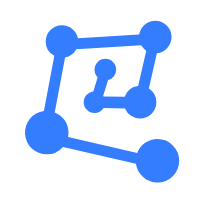Machine Learning Platform For AI

An end-to-end platform that provides various machine learning algorithms to meet your data mining and analysis requirements.

• ##Epidemic Prediction Solution

This technology can be used to predict the spread of COVID-19 and help decision makers evaluate the impact of various prevention and control measures on the development of the epidemic.

• ##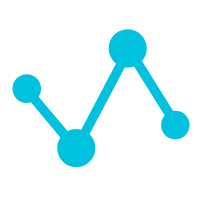Machine Translation

Relying on Alibaba's leading natural language processing and deep learning technology.

• ##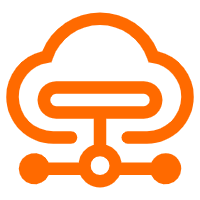Starter Package

High-performance virtual machines with data transfer plan, starting from \$2.50 per month